Related Articles
Find tags by CSS class using BeautifulSoup
• Last Updated : 16 Mar, 2021

In this article, we will discuss how to find tags by CSS using BeautifulSoup. We are given an HTML document, we need to find and extract tags from the document using the CSS class.

Examples:

```HTML Document:
<html>
<title> Geeksforgeeks </title>
<body>
<div class="ext" >Extract this tag</div>
</body>
</html>

Output:
<div class="ext" >Extract this tag</div>```

Required Modules:

• bs4: It is a python library used to scrape data from HTML, XML, and other markup languages.
Make sure you have pip installed on your system.
Run the following command in the terminal to install this library-
```pip install bs4
or
pip install beautifulsoup4```

Approach:

• Import bs4 library
• Create an HTML doc
• Parse the content into a BeautifulSoup object
• Searching by CSS class –  The name of the CSS attribute, “class”, is a reserved word in Python. The compiler gives syntax error if class is used as a keyword argument. We can search CSS class using the keyword argument class_
We can pass class_ a string, a regular expression, a function, or True.
• find_all() with keyword argument class_ is used to find all the tags with the given CSS class
If we need to find only one tag then, find() is used
• Print the extracted tags.

Example 1: Find the tag using find() method

## Python3

 `# Import Module``from` `bs4 ``import` `BeautifulSoup`` ` `# HTML Document``HTML_DOC ``=` `"""``              ````               ````                   `` Geeksforgeeks ``               ````               ````                   ``
Extract this tag
``               ````             ````            ``"""`` ` `# Function to find tags``def` `find_tags_from_class(html):`` ` `    ``# parse html content``    ``soup ``=` `BeautifulSoup(html, ``"html.parser"``)`` ` `    ``# find tags by CSS class``    ``div ``=` `soup.find(``"div"``, ``class_``=` `"ext"``)`` ` `    ``# Print the extracted tag``    ``print``(div)`` ` `# Function Call``find_tags_from_class(HTML_DOC)`

Output: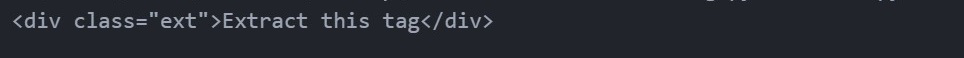Example 2: Find all the tags using find_all() method

## Python3

 `# Import Module``from` `bs4 ``import` `BeautifulSoup`` ` `# HTML Document``HTML_DOC ``=` `"""``              ````               ````                   `` Table Data ``               ````               ````                ````                   ``
This is row 1 This is row 2 This is row 3 This is row 4 This is row 5
``                    ````                    ````                    ````                    ````                    ````                   ````                ````               ````             ````            ``"""`` ` `# Function to find tags``def` `find_tags_from_class(html):`` ` `    ``# parse html content``    ``soup ``=` `BeautifulSoup(html, ``"html.parser"``)`` ` `    ``# find tags by CSS class``    ``rows ``=` `soup.find_all(``"td"``, ``class_``=` `"table-row"``)`` ` `    ``# Print the extracted tag``    ``for` `row ``in` `rows:``        ``print``(row)`` ` `# Function Call``find_tags_from_class(HTML_DOC)`

Output: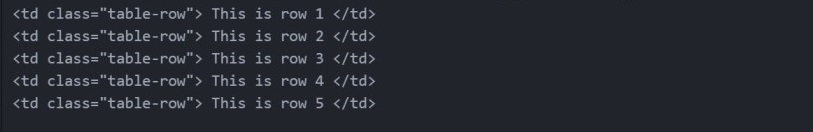Example 3: Finding tags by CSS class using Regular Expressions.

## Python3

 `# Import Module``from` `bs4 ``import` `BeautifulSoup``import` `re`` ` `# HTML Document``HTML_DOC ``=` `"""``              ````               ````                   `` Table Data ``               ````               ````                ````                   ``
This is row 1 This is row 2 This is row 3 This is row 4 This is row 5
``                    ````                    ````                    ````                    ````                    ````                   ````                ````               ````             ````            ``"""`` ` `# Function to find tags``def` `find_tags_from_class(html):`` ` `    ``# parse html content``    ``soup ``=` `BeautifulSoup(html, ``"html.parser"``)`` ` `    ``# find tags by CSS class using regular expressions``    ``# \$ is used to match pattern ending with``    ``# Here we are finding class that ends with "row"``    ``rows ``=` `soup.find_all(``"td"``, ``class_``=` `re.``compile``(``"row\$"``))`` ` `    ``# Print the extracted tag``    ``for` `row ``in` `rows:``        ``print``(row)`` ` `# Function Call``find_tags_from_class(HTML_DOC)`

Output: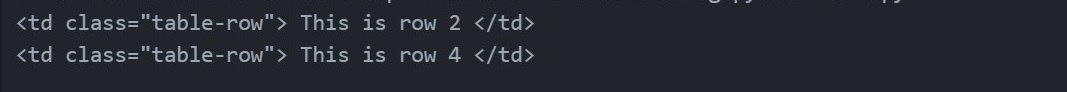Explanation:

```<td class="table-row"> This is row 2 </td>
<td class="table-row"> This is row 4 </td>```

Above two tags class name ends with “row”. Therefore, they are extracted. Other tags class name doesn’t end with “row”. Therefore, they are not extracted.

Example 4: Finding tags by CSS class using the user-defined function.

## Python3

 `# Import Module``from` `bs4 ``import` `BeautifulSoup`` ` `# HTML Document``HTML_DOC ``=` `"""``              ````               ````                   `` Table Data ``               ````               ````                ````                   ``
This is invalid because len(table) != 3 This is valid because len(row) == 3 This is invalid because len(data) != 3 This is valid because len(hii) == 3 This is invalid because class is None
``                    ````                    ````                    ````                    ````                    ````                   ````                ````               ````             ````            ``"""`` ` `# Returns true if the css_class is not None``# and length of css_class is equal to 3``# else returns false``def` `has_three_characters(css_class):``    ``return` `css_class ``is` `not` `None` `and` `len``(css_class) ``=``=` `3`` ` ` ` `# Function to find tags``def` `find_tags_from_class(html):`` ` `    ``# parse html content``    ``soup ``=` `BeautifulSoup(html, ``"html.parser"``)`` ` `    ``# find tags by CSS class using user-defined function``    ``rows ``=` `soup.find_all(``"td"``, ``class_``=` `has_three_characters)`` ` `    ``# Print the extracted tag``    ``for` `row ``in` `rows:``        ``print``(row)`` ` `# Function Call``find_tags_from_class(HTML_DOC)`

Output: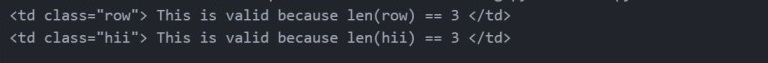Example 5: Finding tags by CSS class from a website

## Python3

 `# Import Module``from` `bs4 ``import` `BeautifulSoup``import` `requests`` ` `# Assing website``import` `requests ``URL ``=` `"https://www.geeksforgeeks.org/"``HTML_DOC ``=` `requests.get(URL)`` ` `# Function to find tags``def` `find_tags_from_class(html):`` ` `    ``# parse html content``    ``soup ``=` `BeautifulSoup(html.content, ``"html5lib"``)`` ` `    ``# find tags by CSS class``    ``div ``=` `soup.find(``"div"``, ``class_``=` `"article--container_content"``)`` ` `    ``# Print the extracted tag``    ``print``(div)`` ` `# Function Call``find_tags_from_class(HTML_DOC)`

Output: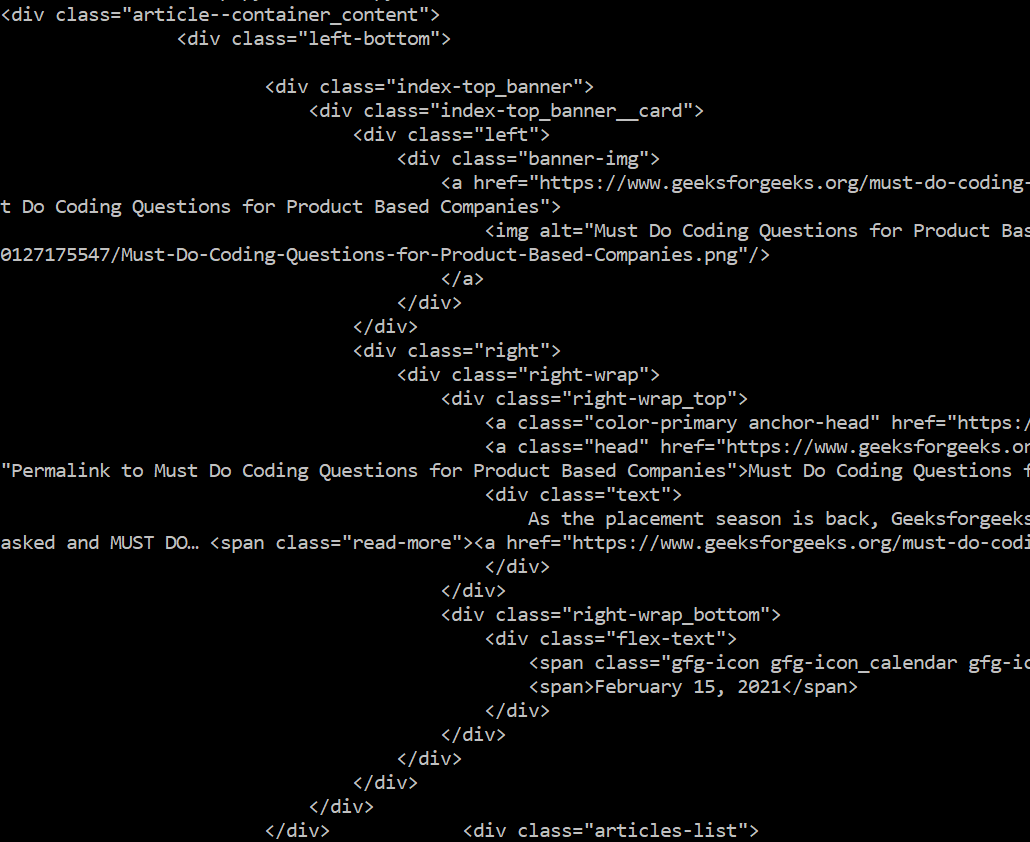Attention geek! Strengthen your foundations with the Python Programming Foundation Course and learn the basics.

To begin with, your interview preparations Enhance your Data Structures concepts with the Python DS Course. And to begin with your Machine Learning Journey, join the Machine Learning – Basic Level Course

My Personal Notes arrow_drop_up